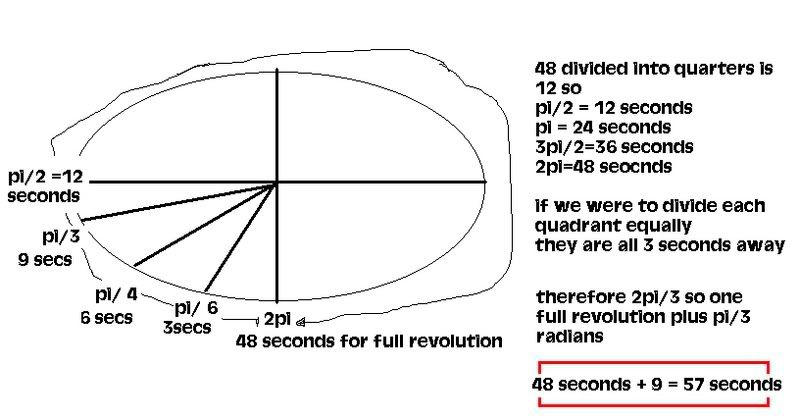• This workspace has been inactive for over 11 months, and is scheduled to be reclaimed. Make an edit or click here to mark it as active.
• If you are citizen of an European Union member nation, you may not use this service unless you are at least 16 years old.

• Whenever you search in PBworks or on the Web, Dokkio Sidebar (from the makers of PBworks) will run the same search in your Drive, Dropbox, OneDrive, Gmail, Slack, and browsed web pages. Now you can find what you're looking for wherever it lives. Try Dokkio Sidebar for free.

View

# CircularFunctions

last edited by 16 years, 4 months ago

# Problem 1 of 3

A ferris wheel at an amusement park has riders get on at the bottom of the wheel which is 3 meters above the ground. The highest point of the ride is 21 meters above the ground and it takes 48 seconds to make a one complete revolution.

(a) Write two equations, one sine and one cosine, that models the height of a rider over time beginning from when they first start the ride.

(b) How long would it take a rider to pass through an angle of 2π/3 radians?

(c) How long during each revolution is a rider higher than 10 meters above the ground?

Solution

a) H(t)= 3sin[(2π/48(t+0)]+12 and H(t)= -3cos[(2π/48)(t-12)]+12b) it would take about 9 + 48 = 57 seconds to pass through the angle of 2π/3 radiansc) 10 = 3sin[(2π/48(t)]+12

let [2π/48(t)]=x

10=3sinx+12

-2=3sinx

sinx=-2/3

(48/2π)[2π/48(t)]= -2/3(48/2π)

t=-2/3(48/2π)

^^ i think..need my notes and calculator

still working on this...don't take..hehe sorry got no calculator on me right now..ill be back tomorrow =D

# Problem 2 of 3

Determine the general solution algebraically. (Solve over the set of real numbers and write your answer accurate to at least 3 decimal places.)

3cos2 x = 8cos x - 4

Solution

# Problem 3 of 3

A sinusoidal curve has a minimum point at (-π/3, -5) and the closest maximum point to the right is (π/6, 3).

(a) Determine an equation of this curve.

(b) Sketch the graph.

Solution